Next: Normal Shocks Up: One-Dimensional Compressible Inviscid Flow Previous: Mach Number

# Sonic Flow through a Nozzle

Consider an ideal gas flowing steadily through a straight nozzle with a slowly-varying cross-sectional area,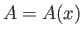, wheremeasures distance along the nozzle. The temperature,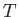, density,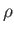, pressure,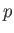, and normal velocity,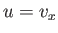, are all assumed to be slowly-varying functions ofthat are constant across any cross section. Thus, in this quasi-one-dimensional problem, we are effectively disregarding the comparatively small components of the flow velocity orthogonal to the-axis. Furthermore, because all streamlines have the same parameter values in any cross section, the flow is homentropic.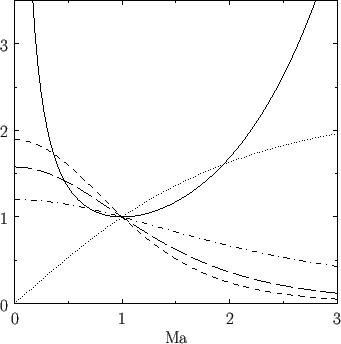Of course, the net mass flow rate,, must be constant along the nozzle, so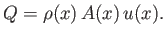(14.69)

In particular,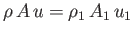, where the subscriptrefers to the sonic point (assuming that such a point exists). Using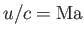and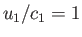, we find that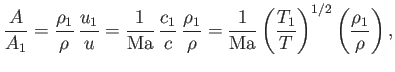(14.70)

where use has been made of Equation (14.45). It follows from Equations (14.66) and (14.68) that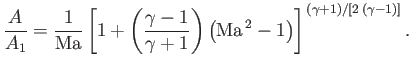(14.71)

Moreover, the previous two equations can be combined with Equation (14.68) to give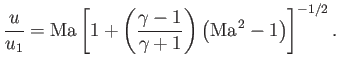(14.72)

Figure 14.1 shows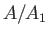,,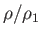,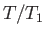, and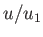, plotted as functions of the local Mach number,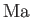, for an ideal gas with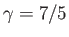. Here, use has been made of Equations (14.71), (14.67), (14.68), (14.66), and (14.72), respectively. Inspecting the curves, we can see, somewhat surprisingly, that the cross-sectional area function,, attains a minimum value when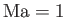. In fact, the figure indicates that, for subsonic flow (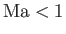), a decreasing cross-sectional area of the nozzle in the direction of the gas flow leads to an increasing flow speed, and decreasing pressure, density, and temperature. However, for supersonic flow (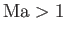), this behavior is reversed, and an increasing cross-sectional area of the nozzle leads to an increasing flow speed, and decreasing pressure, density, and temperature. We conclude that local Mach number of gas flowing through a converging nozzle (i.e., a nozzle whose cross-sectional area decreases monotonically in the direction of the gas flow) can never exceed unity. Moreover, the maximum Mach number (i.e., unity) is achieved on exit from the nozzle, where the cross-sectional area is smallest. On the other hand, the local Mach number of gas flowing through a converging-diverging nozzle (i.e., a nozzle whose cross-sectional area initially decreases in the direction of the gas flow, attains a minimum value, and then increases) can exceed unity. For this to happen, the flow conditions must be arranged such that the sonic point corresponds precisely to the narrowest point of the nozzle, which is generally known as the throat. In this case, as the gas flows through the converging part of the nozzle, the local cross-sectional area,, travels down the left-hand, subsonic branch of thecurve shown in Figure 14.1, while the flow speed,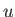, simultaneously increases. After passing through the throat at the sonic speed, the gas flows through the diverging part of the nozzle, and the cross-sectional area travels up the right-hand, supersonic branch of thecurve, while the flow speed continues to increase. The type of converging-diverging nozzle just described is known as a de Laval nozzle, after its inventor, Gustaf de Laval (1845-1913).

Consider a de Laval nozzle whose gas supply is derived from a large reservoir. Assuming that the gas in the reservoir is essentially at rest, it follows that the temperature, pressure, and density of the gas in the reservoir correspond to the stagnation temperature, pressure, and density--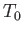,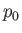, and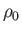, respectively. Equation (14.63)-(14.65) then specify the temperature, pressure, and density of the gas at the throat of the nozzle--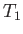,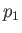, and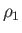, respectively--in terms of the temperature, pressure, and density of the gas in the reservoir.

Suppose that a de Laval nozzle exhausts gas into the atmosphere, whose pressure is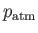. Now, for the case of incompressible flow, the pressure of the gas exhausted from a nozzle,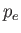(say), must match the ambient pressure,. The reason for this is that any mismatch between the exhaust and ambient pressures is instantly communicated to the whole fluid by means of sound waves that travel infinitely fast (because an incompressible fluid corresponds to the limit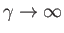). Of course, the sound speed is finite in a compressible gas. However, in subsonic compressible flow, upstream communication is still possible, because the local sound speed exceeds the local flow speed. However, in supersonic compressible flow, upstream communication is impossible, because sound waves cannot catch up with the flow. Consequently, in the case of a nozzle with a subsonic exhaust speed, we would generally expect the exhaust pressure to match the ambient pressure. However, in the case of a nozzle with a supersonic exhaust speed, the exhaust pressure can be significantly different to the ambient pressure.

Equation (14.61) yields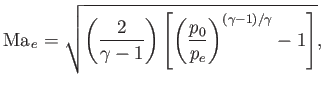(14.73)

where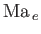is the Mach number of the gas exhausted by the nozzle,the exhaust pressure, andthe reservoir pressure. Suppose that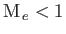--that is, the exhaust speed of the gas is subsonic. In this case, we would expect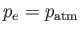, so that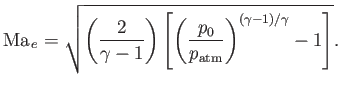(14.74)

It follows that the critical value of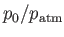at which the exhaust speed becomes sonic (i.e.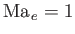) is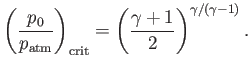(14.75)

Thus, in order for a de Laval nozzle to achieve supersonic exhaust speeds,must exceed this critical value. For the case of a gas with, we find that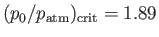. Note that ifdoes not exceed the critical value then, as the gas flows through the converging part of the nozzle, its local cross-sectional area,, travels down the left-hand, subsonic branch of thecurve shown in Figure 14.1. However, when the gas passes through the throat of the nozzle, the area turns around, and then backtracks up the left-hand branch while the gas passes through the diverging part of the nozzle. In this case, the Mach number never reaches unity. In fact, in the converging part of the nozzle, the flow speed increases, while the pressure, density, and temperature decrease. The flow speed attains its maximum, subsonic value at the throat of the nozzle, while the pressure, density, and pressure simultaneously attain minimum values. Finally, in the diverging part of the nozzle, the flow speed decreases, while the pressure, density, and temperature increase.

Equations (14.64) and (14.67) yield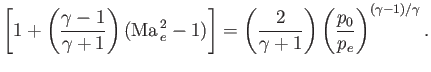(14.76)

Moreover, Equations (14.45) and (14.63) imply that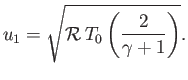(14.77)

Hence, Equations (14.72), (14.73), (14.76), and (14.77) give the following expression for the exhaust speed from a de Laval nozzle,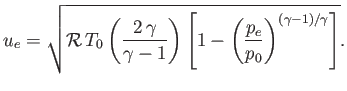(14.78)

Becausecannot be negative, we deduce that there is an upper limit to the exhaust speed: namely,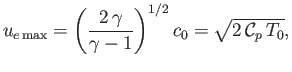(14.79)

where use has been made of Equation (14.60). Here,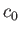is the reservoir sound speed.

If the exhaust pressure of a de Laval nozzle is higher than the ambient pressure,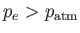, then the gas is said to be under-expanded. In this case, a pattern of standing shock waves, called shock diamonds, forms in the exhaust plume external to the nozzle. On the other hand, if the exhaust pressure is lower than the ambient pressure,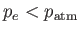, then the gas is said to be over-expanded. In this case, a static shock front forms inside the diverging part of the nozzle. (See Section 14.8.) As the gas passes through the front, its speed drops abruptly from a supersonic to a subsonic value, whereas the pressure, density, and temperature all increase abruptly. As the subsonic gas flows through the remainder of the nozzle, its velocity decreases further.Next: Normal Shocks Up: One-Dimensional Compressible Inviscid Flow Previous: Mach Number
Richard Fitzpatrick 2016-01-22